# Statistically Falsifying a Model

I frequently come across claims that someone has “disproven” a mainstream theory related to climate change. When I look at what has been done, it invariably turns out that the person doing the “disproving” seems to have no understanding of what would be a legitimate procedure for “falsifying” a scientific model.

Instead, they’ve done something else. They did something which might seem convincing to those who don’t understand the scientific method, but what they did doesn’t actually demonstrate ANYTHING about the model or theory allegedly being “tested.”

Maybe some other time I’ll explain how such “tests” seem to go wrong.

However, what I want to do here is sketch how it works when someone is correctly testing a scientific model against data.

This description might not make as much sense as I’d like, out of context. But, I hope it may still have value for some people.

What originally led me to write this up was a conversation I had with someone in which they claimed that data about paleoclimate 300 million years ago “proves” that mainstream theories about climate are wrong. I applied the following procedure to test the planetary temperature formula against paleoclimate data. That analysis showed, given the high level of uncertainty in the data, that the data was consistent with (was not falsified by) the data. The person I was talking to didn’t understand what I was doing. Hence, I wrote the following to conceptually explain the correct procedure for testing a scientific model against a dataset.

If someone claims to be using data to “falsify” a theory and what they are doing doesn’t look anything like what follows, then chances are good that they haven’t “falsified” anything, despite what they might believe.

Here is a general description of how one is supposed to compare a model to data to verify/falsify the model.

Suppose there is a model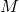which asserts that a quantity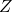is a function of quantities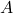,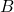,,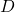,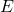:

(1)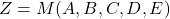Suppose also that you have measured values for these quantities at time, where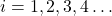You know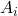,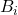,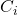,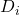,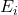,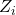for various values of.

You want to assess whether the model is consistent with the data or the data falsifies the model.

The first step in the process is to assume that each measured value reflects an actual value plus a random error term:

(2)where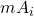is the measured value ofat time,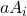is the actual value ofat time, and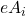is the random measurement error associated with estimatingat time.

To work with this, one needs to make some inferences about the statistical properties of the measurement  errors. Generally one assesses the likely nature of the statistical distribution (e.g., that the errors are likely to obey “Gaussian” statistics) and then use the data to infer the parameters of distribution, e.g., the “standard deviation.” That is what is meant by estimating the “uncertainty” of a measurement.

The model applies to the actual values, not to the measured values. If the model is valid, then:

(3)But, one knows measured values, not actual values. So, when combining the model with the data, one comes up with an equation like this:

(4)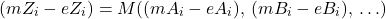That’s the equation that will be true IF the model is valid.

But, how can we verify/falsify the model, given that the “error” terms,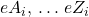are all unknown?

That’s where the next step of the process comes into play: we “fit” the model to the data.  That means that we identify a combination of “error” terms (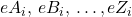) such that the equation is satisfied and the error terms are collectively as small as possible.

Once that has been done, the final step is to assess how improbable the “fit” is.

This involves estimating the probability that the assumed error term values,  (), could have come about by chance, given what is known about the statistical properties of the errors, i.e., given what is known about the measurement uncertainties.

You do an estimation of the probability and come to a conclusion like “the assumed error values could easily have arisen by chance” or “there is only a one in a thousand chance that the error values could have happened at random.” If the former statement applies, the model is consistent with the data. If the latter statement applies, then the model is invalidated, at least within some certain level of “confidence”, e.g., the model failed the test at a confidence level of one in a thousand.

Doing rigorous statistical testing is quite subtle and there are nuances to the process that go way beyond what I’ve described. But, that’s the general idea.

This process critically depends on estimating the “uncertainty” associated with each measurement. If the uncertainties are small, it is comparatively easy for the data to tell us that a model is unlikely to be true. However, if the uncertainties are large, it can be nearly impossible for the data to falsify a model or give us any reliable information on how good or bad a model is.

Any “test” of a theory that doesn’t include both (a) quantitative predictions from the theory under test and (b) careful consideration of “uncertainties” is almost certainly NOT a legitimate test of the the theory.